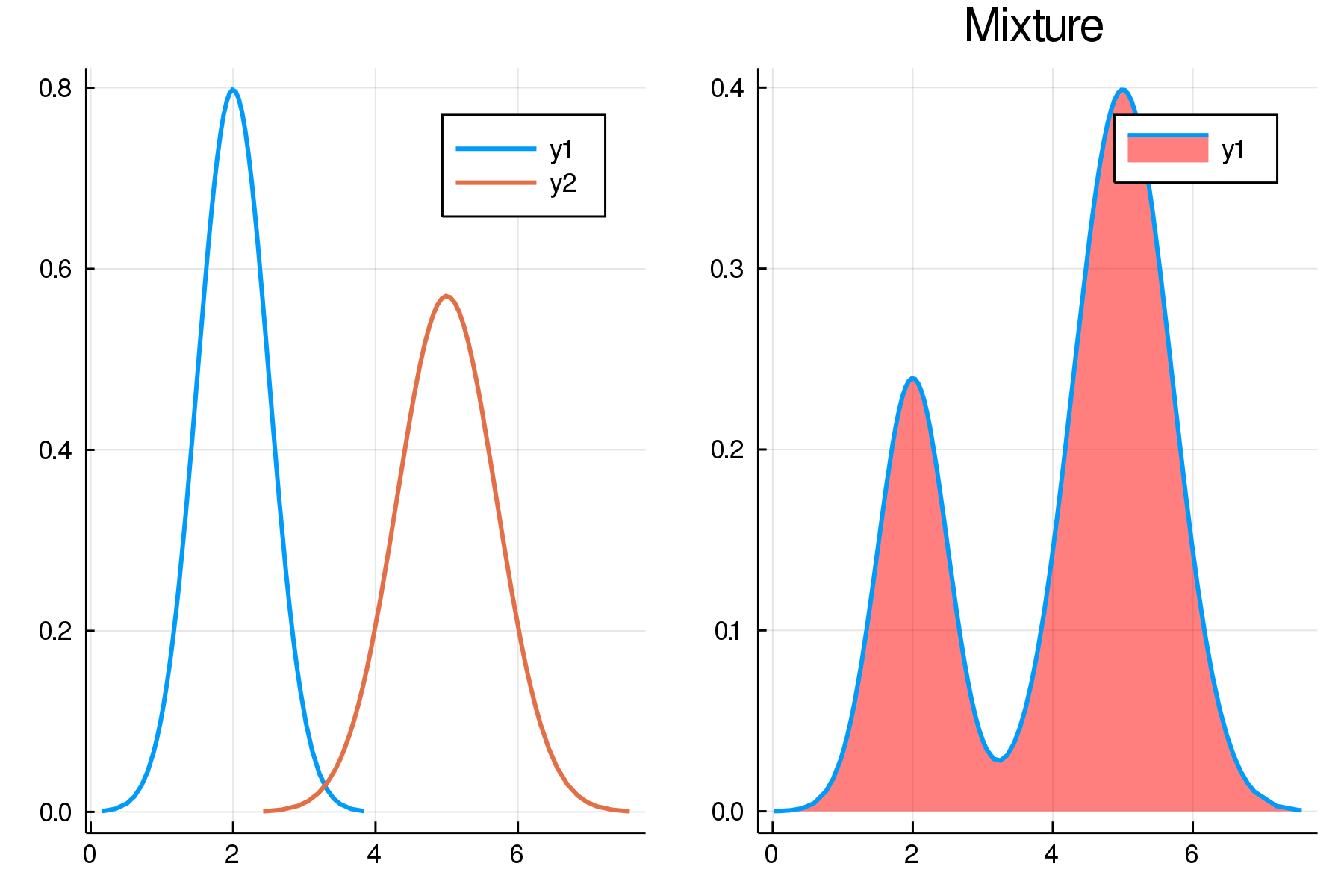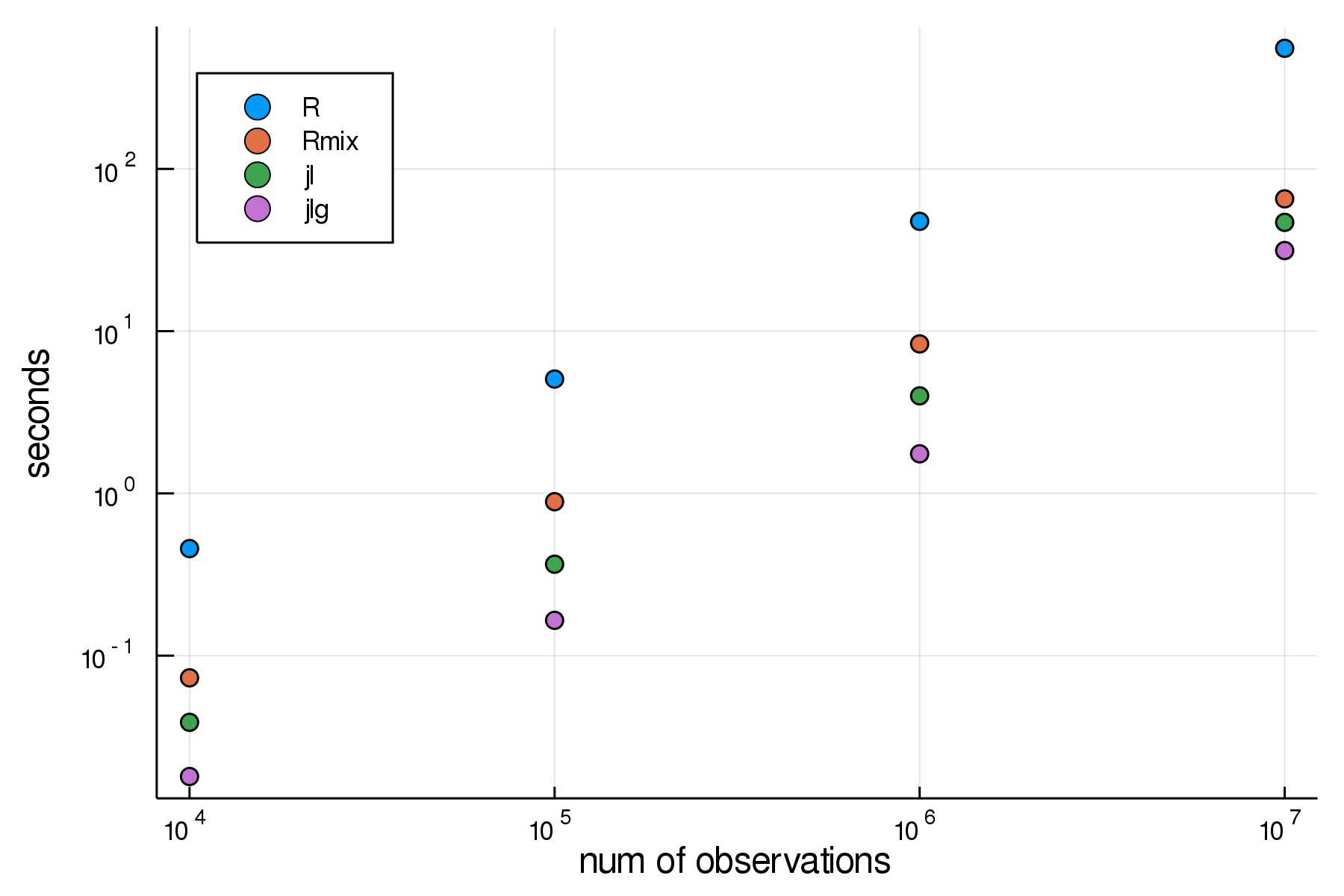# EM Benchmarks

I am getting into estimating mixture models at the moment. In particular in the context of models of wage formation where unobserved heterogeneity stemming from both firm and worker side is often modeled with a mixture model. The main assumptions are that

1. Firms are classifiable into types $l \in \{1,\dots,L\}$, workers into $k \in \{1,\dots,K\}$
2. If Worker $i$ is of type $k$ and works for firm $l$ in a certain period, their wages are drawn from distribution $\mathcal{N}(\mu_{k,l},\sigma_{k,l})$.

This kind of model is at the current frontier of econometrics, and a recent paper is Bonhomme, Lamadon and Manresa (Econometrica 2019), ungated here, with a great replication package in R.

The estimation of such models often involves using the EM-Algorithm, which I won’t describe in detail here.

## Weapon of Choice?

Now before getting to the full model above, I wanted to know my options in terms of programming language. I decided to benchmark a simple version of the above problem: there is only one firm type (all workers at the same firm), and there are only two worker types, $K=2$. I am considering two options in terms of language: R and julia.

I’ll do a hand-coded version in each language, as well as use a package from each:

• Hand-coding is relevant because my final problem will need some modification of existing algorithms.
• Packages are relevant because they often provide efficient implementations, and could be the building block on which my extension is based.

Here goes!

### Benchmark Setup

I will benchmark everything out of a julia session by relying on the RCall.jl package (benchmark code on this repo). RCall.jl launches an R session from within julia and allows to go back and forth with surprisingly little overhead (accesses the same locations in RAM, so no data is copied). The advantage of this is that I can create the exact same benchmarking data to test in both languages. So all code you see here is valid julia, even though sometimes it contains some R. Cool, right?🕺

Here is the data creator:

function sdata(k,n; doplot = false)
Random.seed!(3333)
# true values
μ = [2.0,5.0]
σ = [0.5,0.7]
α = [0.3,0.7]

m = MixtureModel([Normal(μ[i], σ[i]) for i in 1:2], α)
if doplot
plot(
plot(m,linewidth=2),
plot(m,linewidth=2, fill=(0,:red,0.5), components = false, title="Mixture"),dpi = 300
)
savefig("mixtures.png")
end
y = rand(m,n)

return Dict(:y => y, :μ => μ, :σ => σ, :α => α)
end

• sets true mixture parameters
• creates a Distributions.MixtureModel data type
• optionally makes a plot from it
• draws n random realizations from it

The setup looks like this:All benchmarks will now proceed in the same way:

1. Take vector y
2. set (the same) wrong starting values
3. run the EM algorithm for iters iterations to find true values of proportion weights $\alpha$, means $\mu$ and variances $\sigma$ for each component.
4. Notice that the starting values are such that the algorithm never fully recovers the true values. Given it’s the same data, however, each implementation will follow the same path for parameter values and run the same number of iterations (again, none until convergence).

### julia by hand

Here is my relatively naive and just-copy-thy-math implemenation in julia:

function bm_jl(y::Vector{Float64};iters=100)

# poor starting values
μ = [4.0,6.0]
σ = [1.0,1.0]
α = [0.5,0.5]

N = length(y)
K = length(μ)

# initialize objects
L = zeros(N,K)
p = similar(L)

for it in 1:iters

dists = [Normal(μ[ik], σ[ik] ) for ik in 1:K]

# evaluate likelihood for each type
for i in 1:N
for k in 1:K
# Distributions.jl logpdf()
L[i,k] = log(α[k]) + logpdf.(dists[k], y[i])
end
end

# get posterior of each type
p[:,:] = exp.(L .- logsumexp(L))

# with p in hand, update
α[:] .= vec(sum(p,dims=1) ./ N)
μ[:] .= vec(sum(p .* y, dims = 1) ./ sum(p, dims = 1))
σ[:] .= vec(sqrt.(sum(p .* (y .- μ').^2, dims = 1) ./ sum(p, dims = 1)))
end
return Dict(:α => α, :μ => μ, :σ => σ)
end


### GaussianMixtures.jl

Next is a julia package written for this purpose. Here is the relevant part:

function bm_jl_GMM(y::Vector{Float64};iters=100)
gmm = GMM(2,1)  # initialize an empty GMM object
# stick in our starting values
gmm.μ[:,1] .= [4.0;6.0]
gmm.Σ[:,1] .= [1.0;1.0]
gmm.w[:,1] .= [0.5;0.5]

# run em!
em!(gmm,y[:,:],nIter = iters)
return gmm
end


### R not-so-naive by hand

• I tried to vectorize as much as possible here
• Self-imposed rules: no Rcpp
• You can see this uses an R-string, where data values are interpolated with a $ into the R session. # this is a julia function! function bm_R(y;iters=100) # that defines an R-string, sent off to R. r_result = R""" library(tictoc) # define a repeat function spread <- function (A, loc, dims) { if (!(is.array(A))) { A = array(A, dim = c(length(A))) } adims = dim(A) l = length(loc) if (max(loc) > length(dim(A)) + l) { stop("incorrect dimensions in spread") } sdim = c(dim(A), dims) edim = c() oi = 1 ni = length(dim(A)) + 1 for (i in c(1:(length(dim(A)) + l))) { if (i %in% loc) { edim = c(edim, ni) ni = ni + 1 } else { edim = c(edim, oi) oi = oi + 1 } } return(aperm(array(A, dim = sdim), edim)) } # define row-wise logsumexp logRowSumExp <- function(M) { if (is.null(dim(M))) {return(M)} vms = apply(M,1,max) log(rowSums(exp(M-spread(vms,2,dim(M))))) + vms } # define the function to be timed in R simpleEM <- function(y,iters){ K = 2 N = length($y)

EMfun <- function(mu,sigma,alpha,iters){
# allocate arrays
p = array(0,c(N,K))
L = array(0,c(N,K))

for (it in 1:iters){
# E step

# vectorized over N loop
for (k in 1:K){
L[ ,k] = log(alpha[k]) + dnorm(y,mean = mu[k], sd = sigma[k], log = TRUE)
}
p = exp(L - logRowSumExp(L))

# M step
alpha = colMeans(p)
mu = colSums(p * y) / colSums(p)
sigma = sqrt( colSums( p * (y - spread(mu,1,N))^2 ) / colSums(p) )
}
o =list(alpha=alpha,mu=mu,sigma=sigma)
return(o)
}

# starting values
mu_    = c(4.0,6.0)
sigma_ = c(1.0,1.0)
alpha_ = c(0.5,0.5)

# take time
tic()
out = EMfun(mu_,sigma_,alpha_,iters)
tt = toc()
return(list(result = out, time = tt$toc - tt$tic))
}
simpleEM($y,$iters)   # run function in R!
"""
return r_result
end


### R mixtools package

The mixtools package is a very mature and highly optimized package for EM estimation. Most of the computationally intensive parts are written in C1. My call:

function bm_R_mixtools(y::Vector{Float64};iters=100)

r_result = R"""

library(tictoc)
library(mixtools)

mu    = c(4.0,6.0)
sigma = c(1.0,1.0)
alpha = c(0.5,0.5)

y = $y N = length(y) K = 2 iters =$iters

tic()
result = normalmixEM(y,k = K,lambda = alpha, mu = mu, sigma = sigma, maxit = iters)
tt = toc()
list(result = result, time = tt$toc - tt$tic)

"""
return r_result
end


## Results!

• I use the BenchmarkTools.jl package to benchmark the julia functions. This runs the functions multiple times to account for system noise. (running multiple times also gets rid of any JIT-related delays in julia)
• The R functions are timed within the R process using the tictoc package, so even if there were any significant overhead from RCall.jl, the measurement is immune to that.

Here is the output table, with n for sample size, and times in seconds:

n jl jlg R Rmix
10000 0.0388268 0.0179957 0.457 0.073
100000 0.366047 0.16506 5.068 0.889
1000000 3.99279 1.75384 47.522 8.344
10000000 46.833 31.4021 553.783 65.379

And a much clearer picture using log scales:## Conclusions

1. The naively hand-written code in julia performs very well.
2. The GaussianMixtures.jl performs best throughout
3. Both julia implementations outperform the C-optimized R mixtools package.
4. The vectorized R version comes in slowest.

I take from this that focusing on extending the work in GaussianMixtures.jl for my purposes is the most promising avenue here.

## Code and Versions

Code is on github with full package version manifest.

julia> versioninfo()
Julia Version 1.1.0
Commit 80516ca202 (2019-01-21 21:24 UTC)
Platform Info:
OS: macOS (x86_64-apple-darwin14.5.0)
CPU: Intel(R) Core(TM) i5-5257U CPU @ 2.70GHz
WORD_SIZE: 64
LIBM: libopenlibm

shell> R --version
R version 3.5.1 (2018-07-02) -- "Feather Spray"
Copyright (C) 2018 The R Foundation for Statistical Computing
Platform: x86_64-apple-darwin15.6.0 (64-bit)

R is free software and comes with ABSOLUTELY NO WARRANTY.
You are welcome to redistribute it under the terms of the
GNU General Public License versions 2 or 3.

1. For example, in the package source, look for src/normpost.c which evaluates the matrix of posterior probabilities, object p in the julia code above. ^